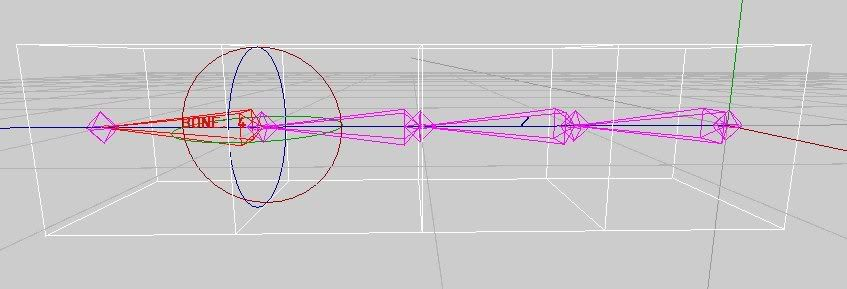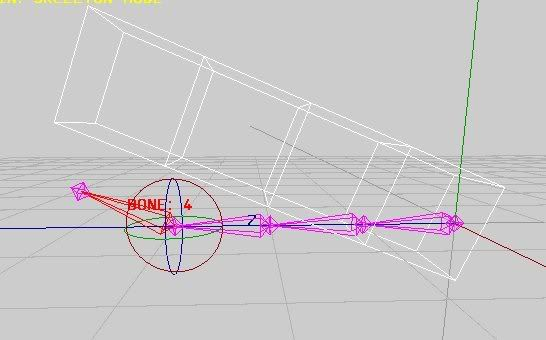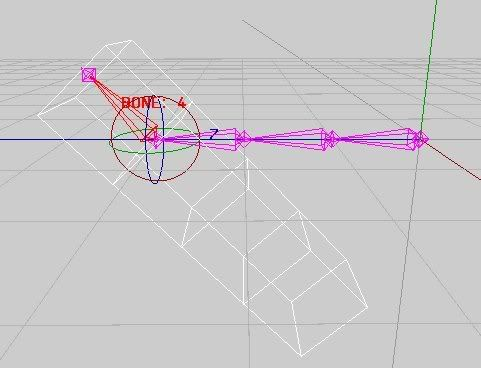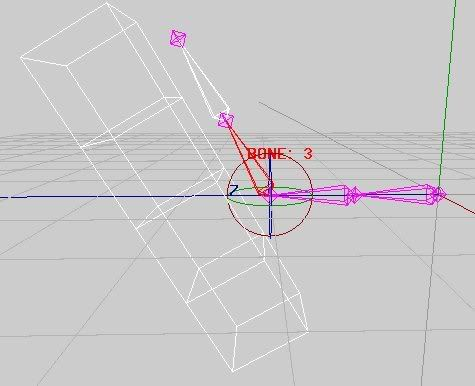# Deforming a mesh with a skeletal structure?

Hi all,

Has anyone got any links to good references on deforming a mesh with a skeletal structure?

I really can’t figure it out…

I have a basic structure allowing me to assign multiple bones to a vertex and specifying the influence for each, something like this:

``````typedef struct {
...
float s_pos;  /* The base postion of the bone */
float e_pos;  /* The end position of the bone */
float matrix;  /* Rotation matrix */
} Bone;

typedef struct {
...
float v;  /* Original vertex position */
float av;  /* Final vertex position */
int num_bones;  /* Number of bones assigned to this vertex */
float *influence;  /* Array of floats indicating influence setting for each attached bone */
Bone **bone;  /* Array of pointers to attached bones */
} Vertex;
``````

Each bone is drawn by translating to it’s s_pos
and transforming by it’s rotation matrix like:

``````glPushMatrix();
glTranslate(bone->s_pos, bone->s_pos, bone->s_pos);
glMultMatrixf(bone->matrix);
/* .. draw bone graphic here .. */
glPopMatrix();
``````

Now the part that’s stumping me is how precisely to calculate the vertice’s positons based on bone assignments?
Each vertex contains the original position (as loaded from a static mesh model) in vertex->v, I need to calculate the deform and place it in vertex->av …

The method I’ve been trying is like this:

``````float tv; /* Temporary vertex positional data */
Bone *bone;
float influence;

for (i = 0; i < 3; i++) vertex->av[i] = vertex->v[i];  /* Set the vertex to the intial position */

for (b = 0; b < vertex->num_bones; b++) {
bone = vertex->bone[b];  /* Get current bone */
influence = vertex->influence[b];  /* Get influence of current bone */

/* Set tv to original vertex position and subtract bone base position - so that rotation happens about the bones axis and not the origin */
for (i = 0; i < 3; i++) tv[i] = vertex->v[i] - bone->s_pos[a];

mult_vector_by_matrix(tv, bone->matrix);  /* Transform tv by the bone's rotation matrix */

for (i = 0; i < 3; i++) {
tv[i] += bone->pos[i];  /* Add bone's base position back into tv */

vertex->v[i] += ((tv[i] - vertex->v[i]) * influence);  /* Calculate offest to orginal position and multiply by influence */
}
}
``````

I found this method somewhere on the web, it works partly - if I attach a vertex to a bone and rotate only that bone it works right … the minute I rotate a bone higher in the hierarchy (i.e. that bones parent) it goes all wrong…Sorry for the rather long ramble…Thanks!

Each bone has a matrix.

Each matrix is the absolute animation transform for that bone, no nesting other than overall character position and that’s not the issue.

Each vertex is associated with one or more bones and has a weight for each bone it is associated with.

Transform each vertex through each vertex through each of its bone matrices. Then multiply the result by the vertex weight for that bone. Add the final values together for the skinned vertex location.

Basically most vertices will have one bone and a weight of 1.0. Joint vertices will usually have more, typically two and the weights will depend on the proximity of the vertex to one bone or the other.

What you often end up with is the equivalent of rigid body animation for everything except the vertices near the joints which interpolate the results of the bones they connect.

Hi,
Thanks for the replyI’m not sure if I’m understanding you correctly but this is what I tried:

``````float tv; /* Temporary vertex positional data */
Bone *bone;
float influence;

for (i = 0; i < 3; i++) vertex->av[i] = 0.0;  /* Initialise the vertex to 0 */

for (b = 0; b < vertex->num_bones; b++) {
bone = vertex->bone[b];  /* Get current bone */
influence = vertex->influence[b];  /* Get influence of current bone */

/* Set tv to original vertex position */
for (i = 0; i < 3; i++) tv[i] = vertex->v[i];

mult_vector_by_matrix(tv, bone->matrix);  /* Transform tv by the bone's rotation matrix */

/* Append the transformed value back into the final vertex posision */
for (i = 0; i < 3; i++) vertex->v[i] += (tv[i]  * influence);
}
``````

I’m still not getting correct results however, I though it best to use some screenshots to display my problem… (I hope it’s OK to display images)

Here I have a simple square “tube” with four bones running through the exact center, all vertices are connected 100% (influence value = 1.0) to the fourth bone - the selected bone.Using the above code when rotating the selected bone I get the following result: (Obviously not correct)I figured the problem simple enough, it’s rotating about the origin instead of the bones axis, as in my earlier example I tried to fix this by subtracting the bones base position before transforming and adding it back afterwards… this yields:Now that is correct, the way it should be… but I still experience the ealier problem when rotating a parent bone - like such:It goes off for some reasonBy the way, this is an open source Linux based project I’ve started so if anyone feels up to peeking at the sources check out:
http://kudu.sourceforge.net/
But you’d have to do a CVS checkout to get the latest sources, ss the only uploaded release is kind of old and doesn’t have this code at all.

Thanks!